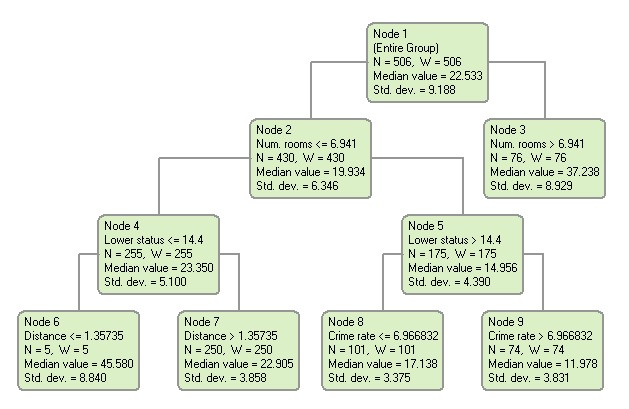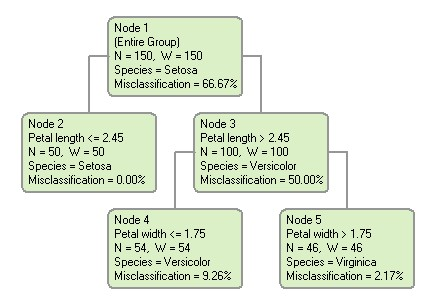# Classification and Regression Trees

DTREG can generate two types of trees depending on whether the target variable is continuous or categorical.

Regression Trees -- If the target variable is continuous, then a regression tree is generated. When using a regression tree to predict the value of the target variable, the mean value of the target variable of the rows falling in a terminal (leaf) node of the tree is the estimated value. An example of a regression tree is shown below. In this example, the target variable is “Median value”. From the tree we see that if the value of the predictor variable “Num. rooms” is greater than 6.941 the estimated (average) value of the target variable is 37.238, whereas if the number of rooms is less than or equal to 6.941 the average value of the target variable is 19.934.Classification Trees -- If the target variable is categorical, then a classification tree is generated. To predict the value (category) of the target variable using a classification tree, use the values of the predictor variables to move through the tree until you reach a terminal (leaf) node, then predict the category shown for that node. An example of a classification tree is shown below. The target variable is “Species”, the species of Iris. We can see from the tree that if the value of the predictor variable “Petal length” is less than or equal to 2.45 the species is Setosa. If the petal length is greater than 2.45, then additional splits are required to classify the species.